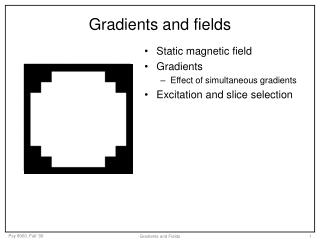DownloadDownload PresentationTélécharger la présentation- - - - - - - - - - - - - - - - - - - - - - - - - - - E N D - - - - - - - - - - - - - - - - - - - - - - - - - - -
##### Presentation Transcript

1. S = 0 S = 0 S = 0 S = 0 S = 0 S = 0 S = 0 S = 0 S = 0 S = 0 S = 0 S = 0 S = 0 S = 0 S = 10 =-10Hz S = 10 =-5Hz S = 10 =0Hz S = 10 =5Hz S = 10 =10Hz S = 0 S = 0 S = 0 S = 0 S = 0 S = 10 =-15Hz S = 10 =-10Hz S = 10 =-5Hz S = 10 =0Hz S = 10 =5Hz S = 10 =10Hz S = 10 =15Hz S = 0 S = 0 S = 0 S = 10 =-20Hz S = 10 =-20Hz S = 10 =-15Hz S = 10 =-10Hz S = 10 =-5Hz S = 10 =0Hz S = 10 =5Hz S = 10 =10Hz S = 10 =15Hz S = 10 =20Hz S = 0 S = 0 S = 10 =-20Hz S = 10 =-15Hz S = 10 =-10Hz S = 10 =-5Hz S = 10 =0Hz S = 10 =5Hz S = 10 =10Hz S = 10 =15Hz S = 10 =20Hz S = 0 S = 0 S = 10 =-20Hz S = 10 =-15Hz S = 10 =-10Hz S = 10 =-5Hz S = 10 =0Hz S = 10 =5Hz S = 10 =10Hz S = 10 =15Hz S = 10 =20Hz S = 0 S = 0 S = 10 =-20Hz S = 10 =-15Hz S = 10 =-10Hz S = 10 =-5Hz S = 10 =0Hz S = 10 =5Hz S = 10 =10Hz S = 10 =15Hz S = 10 =20Hz S = 0 S = 0 S = 0 S = 10 =-15Hz S = 10 =-10Hz S = 10 =-5Hz S = 10 =0Hz S = 10 =5Hz S = 10 =10Hz S = 10 =15Hz S = 0 S = 0 S = 0 S = 0 S = 0 S = 10 =-10Hz S = 10 =-5Hz S = 10 =0Hz S = 10 =5Hz S = 10 =10Hz S = 0 S = 0 S = 0 S = 0 S = 0 S = 0 S = 0 S = 0 S = 0 S = 0 S = 0 S = 0 S = 0 S = 0 Gradients and fields • Static magnetic field • Gradients • Effect of simultaneous gradients • Excitation and slice selection Gradients and Fields

2. Imaging B Gradients and Fields

3. One field, one resonant frequency B RF in RF out Gradients and Fields

4. Field gradient: frequency = localization Bz = (3 + 0.0003z) T Gz = 3 G/cm RF in RF out Gradients and Fields

5. Gradients • Typical strength – 10 to 40 mT/m (1 to 4 G/cm) • Always produce fields aligned with B0 • Typical rise time: 300 microseconds • Risks to subjects: • Peripheral nerve stimulation • Cardiac stimulation http://www.fmrib.ox.ac.uk/~peterj/lectures/hbm_1/sld010.htm Gradients and Fields

6. Z gradient during imaging pulse X gradient during excitation pulse Y gradient during excitation pulse Axial slice Sagittal slice Coronal slice Slice select gradients • Y & Z gradients during imaging pulse • Oblique slice y x z Gradients and Fields

7. Broadband pulse excites fat slice Pulse bandwidth and slice thickness Frequency (Hz) Gradient, e.g. 10 G/cm = 4,260 Hz/cm Position (cm) Gradients and Fields

8. Parts of an RF pulse Carrier (center) frequency BW Frequency (Hz) Gradients and Fields

9. f G = 5.1kHz/cm x Slice selection - edges aren’t exactly hard BW thk = BW/GSS Frequency profile of pulse = spatial profile of slice Gradients and Fields

10. Pulse bandwidth and slice thickness Frequency (Hz) Gradient, e.g. 10 G/cm = 4,260 Hz/cm Narrow pulse excites fat slice Position (cm) Gradients and Fields

11. Pulse bandwidth and slice thickness Frequency (Hz) Gradient, e.g. 10 G/cm = 4,260 Hz/cm Strong gradient decreases slice thickness Position (cm) Gradients and Fields

12. Pulse bandwidth and slice thickness Frequency (Hz) Gradient, e.g. 10 G/cm = 4,260 Hz/cm Center frequency determines slice position Position (cm) Gradients and Fields

13. Slice selection: gradient during pulse Bz = 3T Gz = 3 G/cm RF in RF out Gradients and Fields

14. Pulse sequence diagrams Magnetization preparation Excitation Read-out Nrep RF GSS GPE GRO DAC Gradients and Fields